# Plotting data with matplotlib¶

## Introduction¶

There are many scientific plotting packages. In this chapter we focus on matplotlib, chosen because it is the de facto plotting library and integrates very well with Python.

This is just a short introduction to the `matplotlib` plotting package. Its capabilities and customizations are described at length in the project’s webpage, the Beginner’s Guide, the `matplotlib.pyplot` tutorial, and the `matplotlib.pyplot` documentation. (Check in particular the specific documentation of `pyplot.plot`).

## Basic Usage – `pyplot.plot`¶

Simple use of `matplotlib` is straightforward:

```>>> from matplotlib import pyplot as plt
>>> plt.plot([1,2,3,4])
[<matplotlib.lines.Line2D at 0x7faa8d9ba400>]
>>> plt.show()
```

If you run this code in the interactive Python interpreter, you should get a plot like this:

Two things to note from this plot:

• `pyplot.plot` assumed our single data list to be the y-values;

• in the absence of an x-values list, [0, 1, 2, 3] was used instead.

Note

`pyplot` is commonly used abbreviated as `plt`, just as `numpy` is commonly abbreviated as `np`. The remainder of this chapter uses the abbreviated form.

Note

Enhanced interactive python interpreters such as IPython can automate some of the plotting calls for you. For instance, you can run `%matplotlib` in IPython, after which you no longer need to run `plt.show` everytime when calling `plt.plot`. For simplicity, `plt.show` will also be left out of the remainder of these examples.

If you pass two lists to `plt.plot` you then explicitly set the x values:

```>>> plt.plot([0.1, 0.2, 0.3, 0.4], [1, 2, 3, 4])
```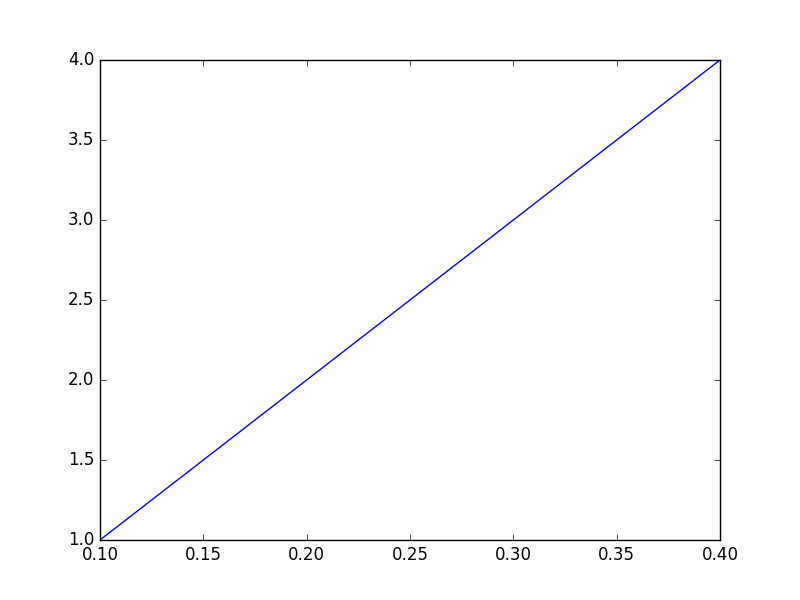Understandably, if you provide two lists their lengths must match:

```>>> plt.plot([0.1, 0.2, 0.3, 0.4], [1, 2, 3, 4, 5])
ValueError: x and y must have same first dimension
```

To plot multiple curves simply call `plt.plot` with as many xy list pairs as needed:

```>>> plt.plot([0.1, 0.2, 0.3, 0.4], [1, 2, 3, 4],
[0.1, 0.2, 0.3, 0.4], [1, 4, 9, 16])
```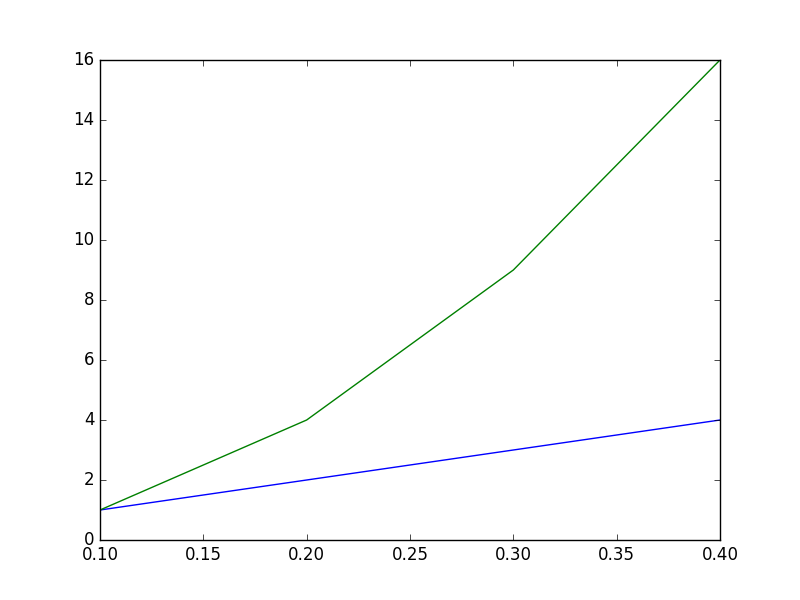Alternaltively, more plots may be added by repeatedly calling `plt.plot`. The following code snippet produces the same plot as the previous code example:

```>>> plt.plot([0.1, 0.2, 0.3, 0.4], [1, 2, 3, 4])
>>> plt.plot([0.1, 0.2, 0.3, 0.4], [1, 4, 9, 16])
```

Adding information to the plot axes is straightforward to do:

```>>> plt.plot([0.1, 0.2, 0.3, 0.4], [1, 2, 3, 4])
>>> plt.plot([0.1, 0.2, 0.3, 0.4], [1, 4, 9, 16])
>>> plt.xlabel("Time (s)")
>>> plt.ylabel("Scale (Bananas)")
```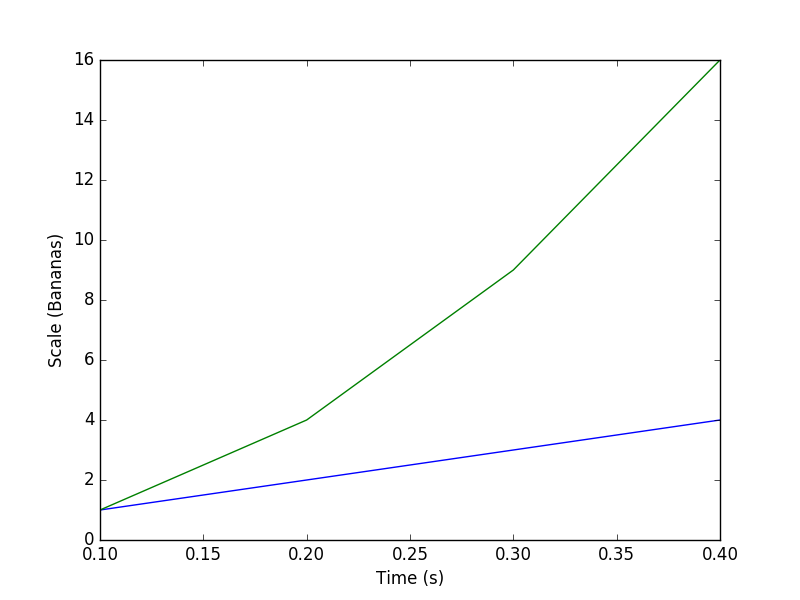Also, adding an legend is rather simple:

```>>> plt.plot([0.1, 0.2, 0.3, 0.4], [1, 2, 3, 4], label='first plot')
>>> plt.plot([0.1, 0.2, 0.3, 0.4], [1, 4, 9, 16], label='second plot')
>>> plt.legend()
```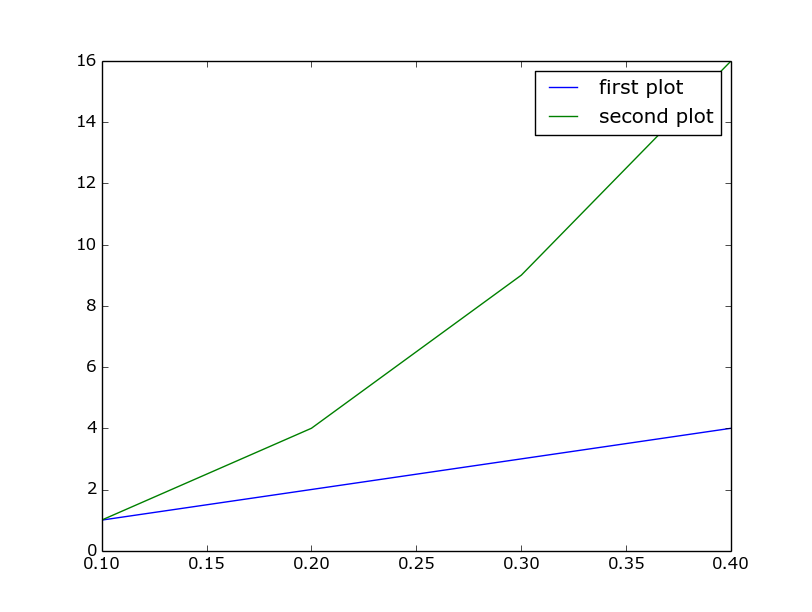And adjusting axis ranges can be done by calling `plt.xlim` and `plt.ylim` with the lower and higher limits for the respective axes.

```>>> plt.plot([0.1, 0.2, 0.3, 0.4], [1, 2, 3, 4])
>>> plt.plot([0.1, 0.2, 0.3, 0.4], [1, 4, 9, 16])
>>> plt.xlabel("Time (s)")
>>> plt.ylabel("Scale (Bananas)")
>>> plt.xlim(0, 1)
>>> plt.ylim(-5, 20)
```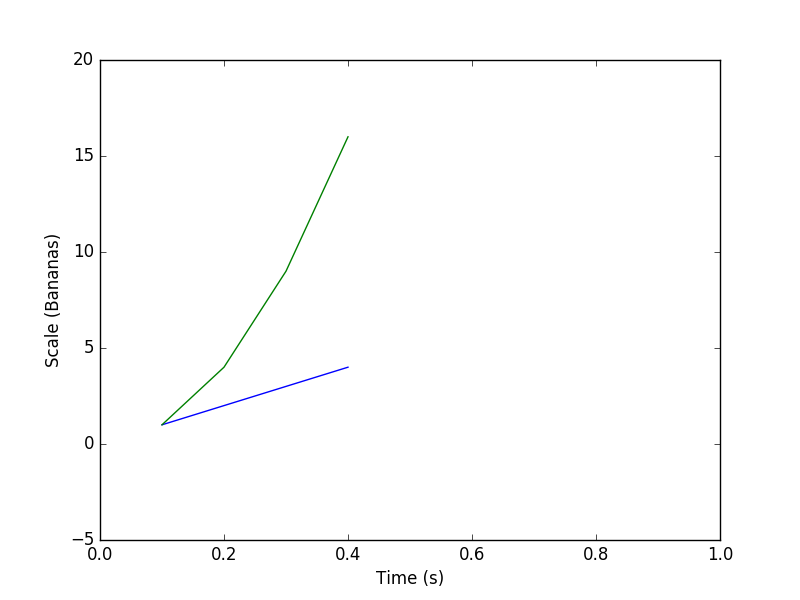In addition to x and y data lists, `plt.plot` can also take strings that define the plotting style:

```>>> plt.plot([0.1, 0.2, 0.3, 0.4], [1, 2, 3, 4], 'rx')
>>> plt.plot([0.1, 0.2, 0.3, 0.4], [1, 4, 9, 16], 'b-.')
>>> plt.xlabel("Time (s)")
>>> plt.ylabel("Scale (Bananas)")
```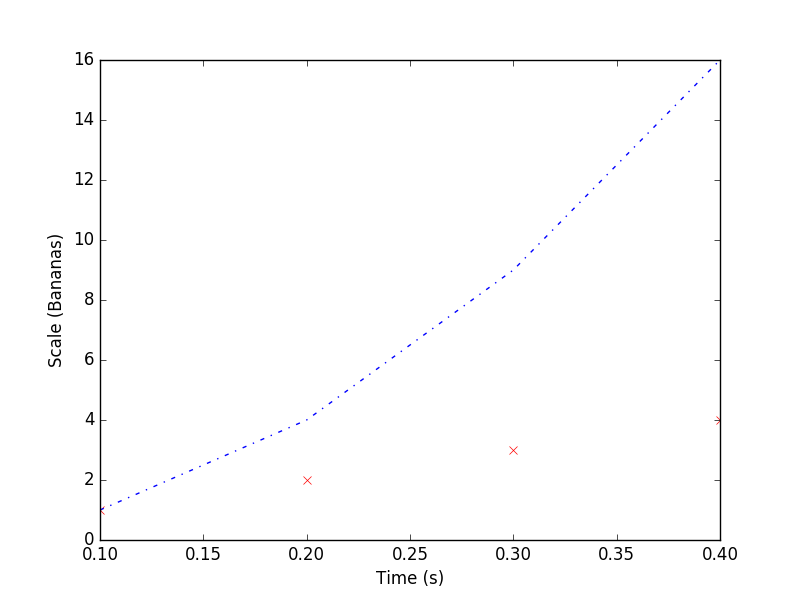The style strings, one per xy pair, specify color and shape: ‘rx’ stands for red crosses, and ‘b-.’ stands for blue dash-point line. Check the documentation of `pyplot.plot` for the list of colors and shapes.

Finally, `plt.plot` can also, conveniently, take numpy arrays as its arguments.

## More plots¶

While `plt.plot` can satisfy basic plotting needs, `matplotlib` provides many more plotting functions. Below we try out the `plt.bar` function, for plotting bar charts. The full list of plotting functions can be found in the the `matplotlib.pyplot` documentation.

Bar charts can be plotted using `plt.bar`, in a similar fashion to `plt.plot`:

```>>> plt.bar(range(7), [1, 2, 3, 4, 3, 2, 1])
```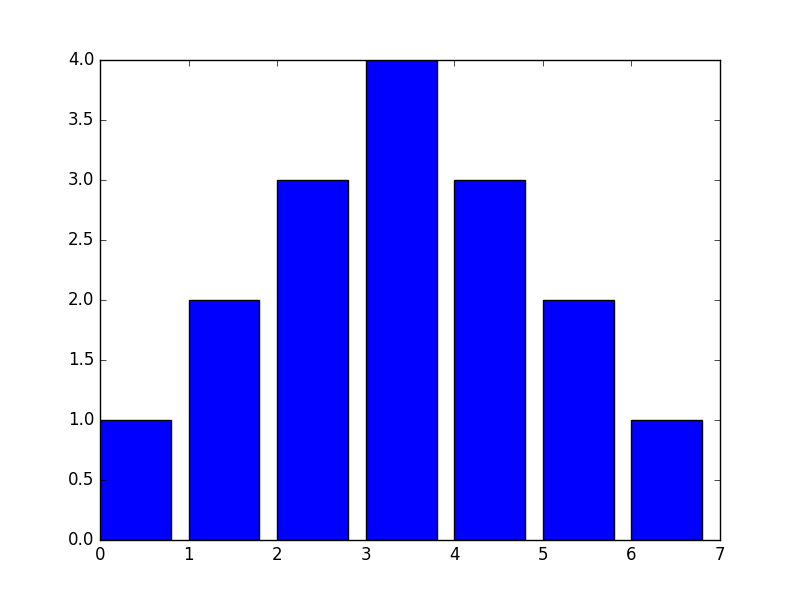Note, however, that contrary to `plt.plot` you must always specify x and y (which correspond, in bar chart terms to the left bin edges and the bar heights). Also note that you can only plot one chart per call. For multiple, overlapping charts you’ll need to call `plt.bar` repeatedly.

One of the optional arguments to `plt.bar` is `width`, which lets you specify the width of the bars. Its default of 0.8 might not be the most suited for all cases, especially when the x values are small:

```>>> plt.bar(numpy.arange(0., 1.4, .2), [1, 2, 3, 4, 3, 2, 1])
```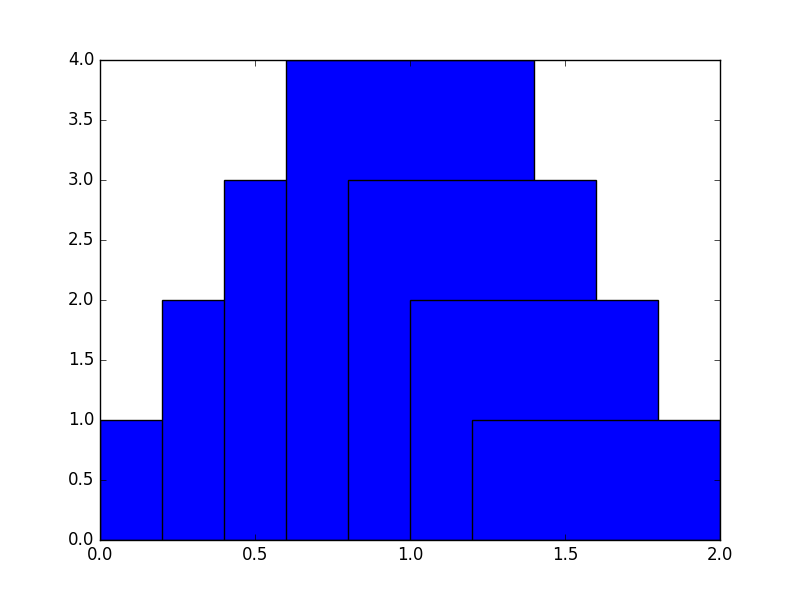Specifying narrower bars gives us a much better result:

```>>> plt.bar(numpy.arange(0., 1.4, .2), [1, 2, 3, 4, 3, 2, 1], width=0.2)
```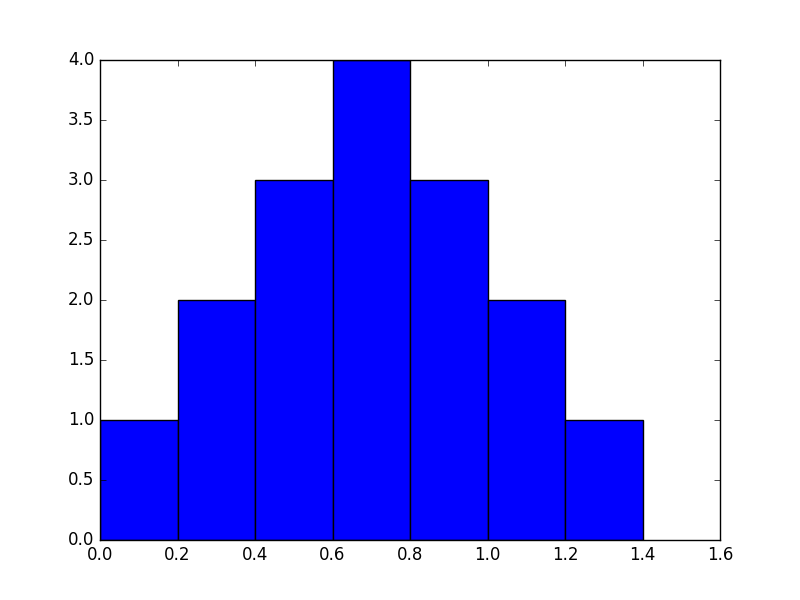Sometimes you will want to compare a function to your measured data; for example when you just fitted a function. Of course this is possible with matplotlib. Let’s say we fitted an quadratic function to the first 10 prime numbers, and want to check how good our fit matches our data.

 ``` 1 2 3 4 5 6 7 8 9 10 11 12 13 14 15 16 17 18 19 20 21``` ```import matplotlib.pyplot as plt def found_fit(x): return 0.388 * x**2 # Found with symfit. x_data = list(range(10)) y_data = [2, 3, 5, 7, 11, 13, 17, 19, 23, 29] x_func = np.linspace(0, 10, 50) # numpy will do the right thing and evaluate found_fit for all elements y_func = found_fit(x_func) # From here the plotting starts plt.scatter(x_data, y_data, c='r', label='data') plt.plot(x_func, y_func, label='\$f(x) = 0.388 x^2\$') plt.xlabel('x') plt.ylabel('y') plt.title('Fitting primes') plt.legend() plt.show() ```

We made the scatter plot red by passing it the keyword argument `c='r'`; `c` stands for colour, `r` for red. In addition, the label we gave to the `plot` statement is in LaTeX format, making it very pretty indeed. It’s not a great fit, but that’s besides the point here.

## Interactivity and saving to file¶

If you tried out the previous examples using a Python/IPython console you probably got for each plot an interactive window. Through the four rightmost buttons in this window you can do a number of actions:

• Pan around the plot area;
• Zoom in and out;
• Access interactive plot size control;
• Save to file.

The three leftmost buttons will allow you to navigate between different plot views, after zooming/panning.

As explained above, saving to file can be easily done from the interactive plot window. However, the need might arise to have your script write a plot directly as an image, and not bring up any interactive window. This is easily done by calling `plt.savefig`:

```>>> plt.plot([0.1, 0.2, 0.3, 0.4], [1, 2, 3, 4], 'rx')
>>> plt.plot([0.1, 0.2, 0.3, 0.4], [1, 4, 9, 16], 'b-.')
>>> plt.xlabel("Time (s)")
>>> plt.ylabel("Scale (Bananas)")
>>> plt.savefig('the_best_plot.pdf')
```

Note

When saving a plot, you’ll want to choose a vector format (either pdf, ps, eps, or svg). These are resolution-independent formats and will yield the best quality, even if printed at very large sizes. Saving as png should be avoided, and saving as jpg should be avoided even more.

## Multiple figures¶

With this groundwork out of the way, we can move on to some more advanced matplotlib use. It is also possible to use it in an object-oriented manner, which allows for more separation between several plots and figures. Let’s say we have two sets of data we want to plot next to eachother, rather than in the same figure. Matplotlib has several layers of organisation: first, there’s an `Figure` object, which basically is the window your plot is drawn in. On top of that, there are `Axes` objects, which are your separate graphs. It is perfectly possible to have multiple (or no) Axes in one Figure. We’ll explain the `add_subplot` method a bit later. For now, it just creates an Axis instance.

 ``` 1 2 3 4 5 6 7 8 9 10 11 12 13``` ```import matplotlib.pyplot as plt x_data = [0.1, 0.2, 0.3, 0.4] y_data = [1, 2, 3, 4] fig = plt.figure() ax = fig.add_subplot(1, 1, 1) ax.plot([0.1, 0.2, 0.3, 0.4], [1, 2, 3, 4]) ax.plot([0.1, 0.2, 0.3, 0.4], [1, 4, 9, 16]) ax.set_xlabel('Time (s)') ax.set_ylabel('Scale (Bananas)') plt.show() ```This example also neatly highlights one of Matplotlib’s shortcomings: the API is highly inconsistent. Where we could do `xlabel()` before, we now need to do `set_xlabel()`. In addition, we can’t show the figures one by one (i.e. `fig.show()`); instead we can only show them all at the same time with `plt.show()`.

Now, we want to make multiple plots next to each other. We do that by calling `plot` on two different axes:

 ``` 1 2 3 4 5 6 7 8 9 10 11 12 13 14 15 16 17 18 19 20 21``` ```x_data1 = [0.1, 0.2, 0.3, 0.4] y_data1 = [1, 2, 3, 4] x_data2 = [0.1, 0.2, 0.3, 0.4] y_data2 = [1, 4, 9, 16] fig = plt.figure() ax1 = fig.add_subplot(1, 2, 1) ax2 = fig.add_subplot(1, 2, 2) ax1.plot(x_data1, y_data1, label='data 1') ax2.plot(x_data2, y_data2, label='data 2') ax1.set_xlabel('Time (s)') ax1.set_ylabel('Scale (Bananas)') ax1.set_title('first data set') ax1.legend() ax2.set_xlabel('Time (s)') ax2.set_ylabel('Scale (Bananas)') ax2.set_title('second data set') ax2.legend() plt.show() ```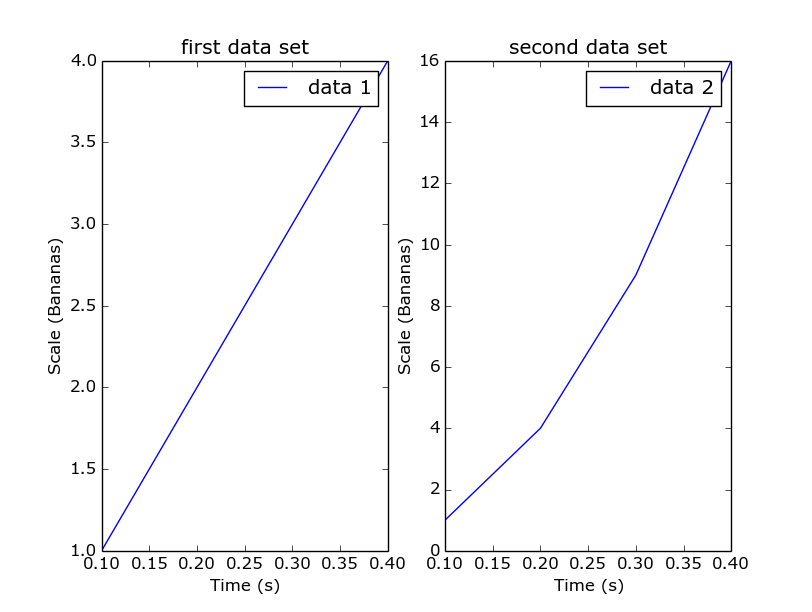The `add_subplot` method returns an `Axis` instance and takes three arguments: the first is the number of rows to create; the second is the number of columns; and the last is which plot number we add right now. So in common usage you will need to call `add_subplot` once for every axis you want to make with the same first two arguments. What would happen if you first ask for one row and two columns, and for two rows and one column in the next call?

## Exercises¶

1. Plot a dashed line.
2. Search the matplotlib documentation, and plot a line with plotmarkers on all it’s datapoints. You can do this with just one call to `plt.plot`.